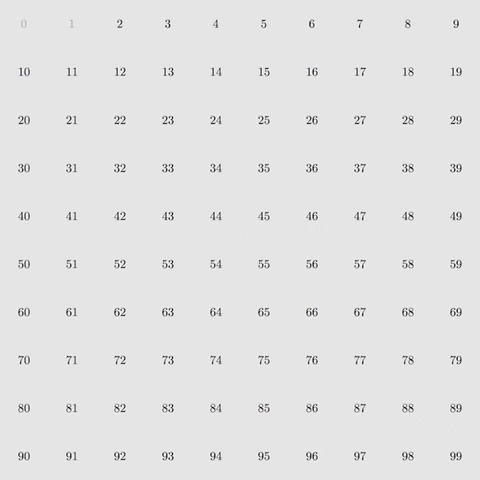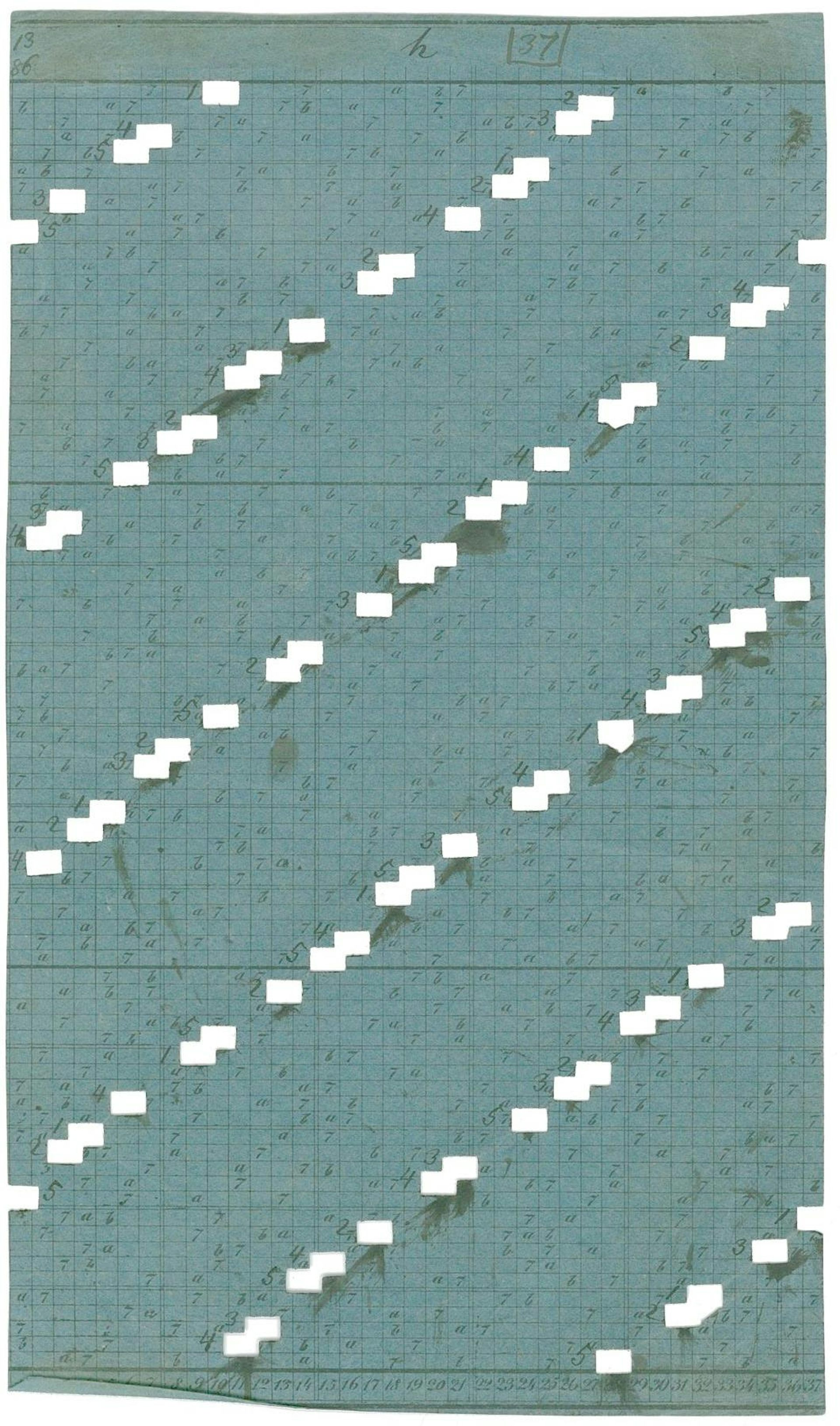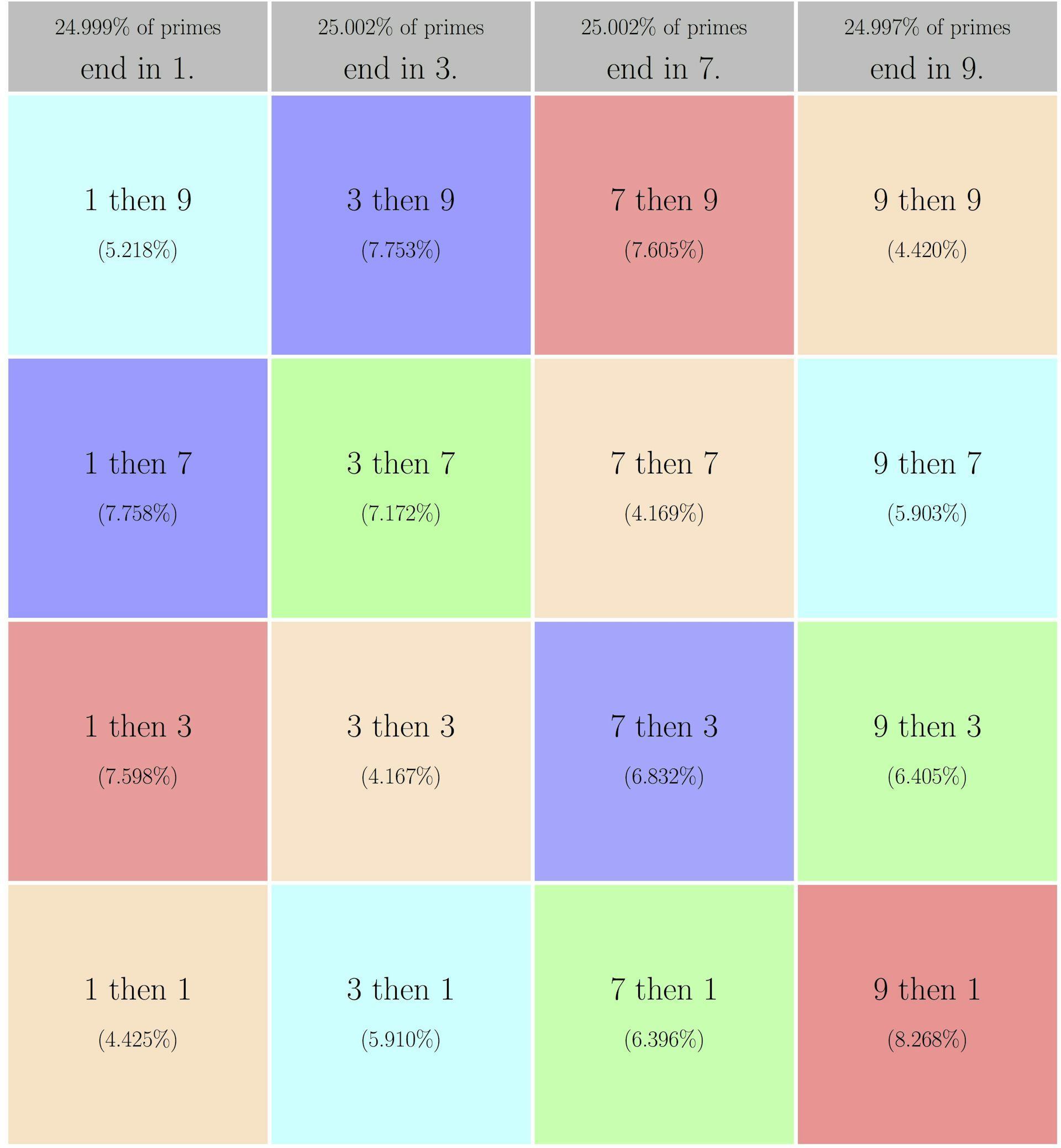# Why prime numbers still fascinate mathematicians, 2,300 years later

On March 20, American-Canadian mathematician Robert Langlands received the Abel Prize, celebrating lifetime achievement in mathematics. Langlands’ research demonstrated how concepts from geometry, algebra and analysis could be brought together by a common link to prime numbers.

When the King of Norway presents the award to Langlands in May, he will honor the latest in a 2,300-year effort to understand prime numbers, arguably the biggest and oldest data set in mathematics.

As a mathematician devoted to this “Langlands program,” I’m fascinated by the history of prime numbers and how recent advances tease out their secrets. Why they have captivated mathematicians for millennia?

## How to find primes

To study primes, mathematicians strain whole numbers through one virtual mesh after another until only primes remain. This sieving process produced tables of millions of primes in the 1800s. It allows today’s computers to find billions of primes in less than a second. But the core idea of the sieve has not changed in over 2,000 years.

“A prime number is that which is measured by the unit alone,” mathematician Euclid wrote in 300 B.C. This means that prime numbers can’t be evenly divided by any smaller number except 1. By convention, mathematicians don’t count 1 itself as a prime number.

Euclid proved the infinitude of primes – they go on forever – but history suggests it was Eratosthenes who gave us the sieve to quickly list the primes.

Here’s the idea of the sieve. First, filter out multiples of 2, then 3, then 5, then 7 – the first four primes. If you do this with all numbers from 2 to 100, only prime numbers will remain.Sieving multiples of 2, 3, 5 and 7 leaves only the primes between 1 and 100. Courtesy of M.H. Weissman.

With eight filtering steps, one can isolate the primes up to 400. With 168 filtering steps, one can isolate the primes up to 1 million. That’s the power of the sieve of Eratosthenes.

## Tables and tables

An early figure in tabulating primes is John Pell, an English mathematician who dedicated himself to creating tables of useful numbers. He was motivated to solve ancient arithmetic problems of Diophantos, but also by a personal quest to organize mathematical truths. Thanks to his efforts, the primes up to 100,000 were widely circulated by the early 1700s. By 1800, independent projects had tabulated the primes up to 1 million.

To automate the tedious sieving steps, a German mathematician named Carl Friedrich Hindenburg used adjustable sliders to stamp out multiples across a whole page of a table at once. Another low-tech but effective approach used stencils to locate the multiples. By the mid-1800s, mathematician Jakob Kulik had embarked on an ambitious project to find all the primes up to 100 million.A stencil used by Kulik to sieve the multiples of 37. AÖAW, Nachlass Kulik, Image courtesy of Denis Roegel, Author provided

This “big data” of the 1800s might have only served as reference table, if Carl Friedrich Gauss hadn’t decided to analyze the primes for their own sake. Armed with a list of primes up to 3 million, Gauss began counting them, one “chiliad,” or group of 1000 units, at a time. He counted the primes up to 1,000, then the primes between 1,000 and 2,000, then between 2,000 and 3,000 and so on.

Gauss discovered that, as he counted higher, the primes gradually become less frequent according to an “inverse-log” law. Gauss’s law doesn’t show exactly how many primes there are, but it gives a pretty good estimate. For example, his law predicts 72 primes between 1,000,000 and 1,001,000. The correct count is 75 primes, about a 4 percent error.

A century after Gauss’ first explorations, his law was proved in the “prime number theorem.” The percent error approaches zero at bigger and bigger ranges of primes. The Riemann hypothesis, a million-dollar prize problem today, also describes how accurate Gauss’ estimate really is.

The prime number theorem and Riemann hypothesis get the attention and the money, but both followed up on earlier, less glamorous data analysis.

## Modern prime mysteries

Today, our data sets come from computer programs rather than hand-cut stencils, but mathematicians are still finding new patterns in primes.

Except for 2 and 5, all prime numbers end in the digit 1, 3, 7 or 9. In the 1800s, it was proven that these possible last digits are equally frequent. In other words, if you look at the primes up to a million, about 25 percent end in 1, 25 percent end in 3, 25 percent end in 7, and 25 percent end in 9.

A few years ago, Stanford number theorists Robert Lemke Oliver and Kannan Soundararajan were caught off guard by quirks in the final digits of primes. An experiment looked at the last digit of a prime, as well as the last digit of the very next prime. For example, the next prime after 23 is 29: One sees a 3 and then a 9 in their last digits. Does one see 3 then 9 more often than 3 then 7, among the last digits of primes?Frequency of last-digit pairs, among successive prime numbers up to 100 million. Matching colors correspond to matching gaps. M.H. Weissman, CC BY

Number theorists expected some variation, but what they found far exceeded expectations. Primes are separated by different gaps; for example, 23 is six numbers away from 29. But 3-then-9 primes like 23 and 29 are far more common than 7-then-3 primes, even though both come from a gap of six.

Mathematicians soon found a plausible explanation. But, when it comes to the study of successive primes, mathematicians are (mostly) limited to data analysis and persuasion. Proofs – mathematicians’ gold standard for explaining why things are true – seem decades away.

### Want to write?

Write an article and join a growing community of more than 94,100 academics and researchers from 3,077 institutions.